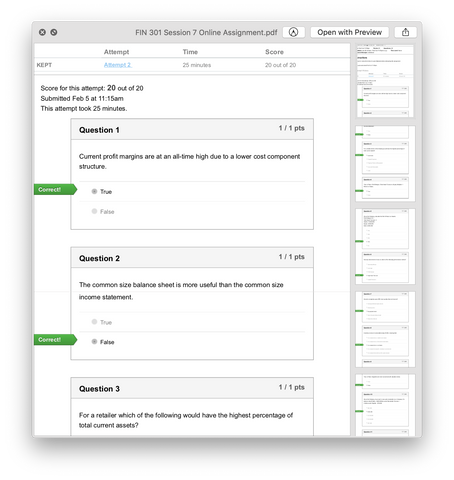# FIN 301 FIN301 Session 7 Online Assignment Answers - PSU

soffix

• \$13.99

FIN 301 Session 7 Online Assignment (Penn State University)

1. Current profit margins are at an all-time high due to a lower cost component structure.
2. The common size balance sheet is more useful than the common size income statement.
3. For a retailer which of the following would have the highest percentage of total current assets?
4. True or False: Profit Margin x Total Asset Turnover x Equity Multiplier = Return on Equity
5. Given the following, calculate the firm's Return on Assets:
6. Grocery stores tend to focus on which of the following performance metrics?
7. How do companies grow EPS more quickly than net income?
8. Inventory turnover is calculated using COGS, meaning that:
9. True or False: Suppliers are most concerned with valuation ratios.
10. Given the following, how much in accounts receivable is on Company X’s balance sheet?Sales - \$400,000Accounts Receivable Turnover – 4.0xAccounts Payable - \$75,000
11. Which of the following is a measure of company’s liquidity?
12. Which of the following is true about common size statements?
13. Given the following information, what is the Profit Margin?
14. Calculate the growth rate of the company's EBIT from 2005 to 2006:
15. Given the following information regarding Angelo’s Pizza Shop’s balance sheet, what is the company’s quick ratio?
16. Which of the following is definitely true?
17. The efficiency of management is best measured by which of the following ratios?
18. Calculate Company PSU's P/E Ratio:
19. Given the following information, what is the Equity Multiplier using the strategic profit model?
20. True or False: Liquidity ratios measure a firm’s ability to meet long-term debt obligations.

Sale

Unavailable

Sold Out